# Difference between revisions of "Butane"

  200 lightgrey butane.pdb  n-butane

Butane (C4H10) (n-butane).

## Models

The NERD parameters are:

 Molecule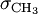$\sigma_{\mathrm {CH}_3}$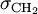$\sigma_{\mathrm {CH}_2}$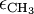$\epsilon_{\mathrm {CH}_3}$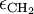$\epsilon_{\mathrm {CH}_2}$ butane 3.91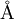$\mathrm{\AA}$ 3.93$\mathrm{\AA}$ 104.0 K 45.8 K

## Isobutane

Isobutane is the branched isomer of butane.

  200 lightgrey isobutane.pdb  Isobutane

## Critical properties

The pressure, temperature and density at the critical point have been calculated for a virial equation of state using the TraPPE-UA force field, and are given in Table I of .

## Virial coefficients

The virial coefficients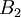$B_2$-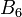$B_6$ as a function of temperature for the TraPPE-UA force field have been tabulated by Schultz and Kofke .